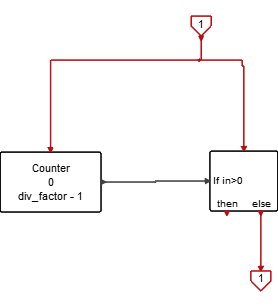# FreqDivider## Diagram Context```if div_factor <= 0
error ('Divider must be greater than 0', 0);
end
if phase < 0 || phase >= div_factor
error ('Phase must be in the range [0 ... Divider - 1]', 0);
end
init = mod (div_factor - phase, div_factor);
```